# Types of tabular charts

In ArcGIS Pro, you can make several types of charts that visualize various characteristics and relationships in tabular data. Tabular data refers to vector or feature data, as well as stand-alone tables.

Learn how to make a chart

 Compare categories and amounts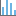Bar chart Use a bar chart to summarize and compare categorical data.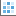Matrix heat chart Use a matrix heat chart to visualize relationships between categorical variables. Explore relationships and correlation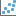Scatter plot Use a scatter plot to visualize the relationship between two numeric variables by displaying one variable on the x-axis and the other variable on the y-axis.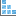Scatter plot matrix Use a scatter plot matrix to create a grid of scatter plots visualizing bivariate relationships between combinations of multiple numeric variables. Visualize distributions and frequency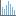Histogram Use a histogram to visualize the distribution of values in a numeric variable by grouping numbers into bins and measuring the frequency of those groups.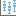Box plot Use a box plot to visualize and compare the distribution and central tendency of numeric values through their quartiles.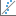QQ plot Use a QQ plot to assess the similarity between the distribution of two numeric variables or between one numeric variable and a standard normal distribution. Visualize change over time or distance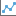Line chart Use a line chart to visualize change over a continuous range, such as time or distance.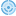Data clock Use a data clock to visualize seasonal or cyclical patterns and trends over time.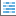Calendar heat chart Use a calendar heat chart to visualize yearly or weekly patterns in temporal data.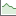Profile graph Use a profile graph to visualize change in the elevation of a 3D polyline.